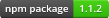# gql

Query language for interpreting genome SNPs

• gql

## Stats

StarsIssuesVersionUpdatedCreatedSize
gql
14211.1.26 years ago9 years ago## Information

 Package gql Description Query language for interpreting genome SNPs Node Version >= 0.4

## Compatibility

This query language is for use with the genomejs JSON format. See the dna2json repository for more information.
Just a reminder: deletions are represented as `-`!

## Usage

This example will create a query that determines if a person is sickle cell effected.
``````var gql = require('gql');
var dna = require('./genome.json');

var query = gql.or([
gql.exact('rs334', 'TT'),
gql.exact('i3003137', 'AA')
]);

var isMatch = query(dna);
console.log(isMatch); // true or false``````

## API

All of these APIs return a boolean-returning function that acts as the truth test. These functions take in one argument, a DNA-JSON object. You can nest as deep as you want, or be as basic as you want.
For example, checking if a person is immune to norovirus is pretty simple:
``````var dna = require('./my-dna.json');

var query = gql.exact('rs601338', 'AA');
var isImmune = query(dna);``````

### Conditions

#### exact(id, genotype)

Evaluates to true if this was the only allele observed.
``````q.exact('rs2032651', 'D'); // will only match genotype D
q.exact('rs2032651', 'AT'); // will only match genotype AT``````

#### has(id, genotype)

Evaluates to true if the allele was observed at all.
``q.has('rs2032651', 'A'); // will match GA, GAT, AB, etc.``

#### exists(id)

Evaluates to true if any allele has been observed.
``q.exists('rs2032651');``

#### doesntExist(id)

Evaluates to true if no allele has been observed.
``q.doesntExist('rs2032651');``

#### not(condition)

Inverts the result of another condition.
``````// evaluates to true if rs2032651 != AA
var query = q.not(q.exact('rs2032651', 'AA'));

query({
rs2032651: {
genotype: 'TT'
}
}); // true

query({
rs2032651: {
genotype: 'AA'
}
}); // false``````

#### only(id, allele)

Evaluates to true if only the allele was observed at the specified position.
``q.only('rs2032651', 'A'); // will match A, AA, AAA, etc.``

### Aggregate Conditions

#### or(conditions...)

Evaluates to true if any of the given condition functions evaluate to true.
``````q.or([
q.has('rs2032651', 'A'),
q.doesntExist('rs2032651')
]);``````

#### and(conditions...)

Evaluates to true if all of the given condition functions evaluate to true.
``````q.and([
q.exists('rs2032652'),
q.has('rs2032651', 'A')
]);``````

#### atLeast(number, conditions...)

Evaluates to true if the number of given condition functions evaluating to true is equal to or greater than the given number.
``````q.atLeast(2, [
q.exists('rs2032652'),
q.has('rs2032651', 'A'),
q.exact('rs2032653', 'AT'),
q.exact('rs2032654', 'GG')
]);``````

#### none(conditions...)

Evaluates to true if all of the given condition functions evaluate to false.
``````q.none([
q.has('rs2032651', 'A'),
q.doesntExist('rs2032651')
]);``````